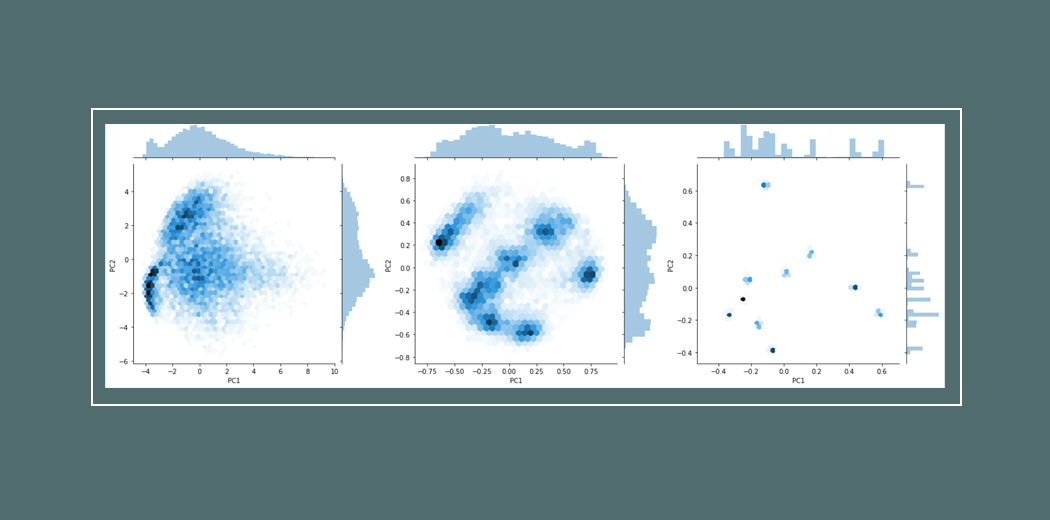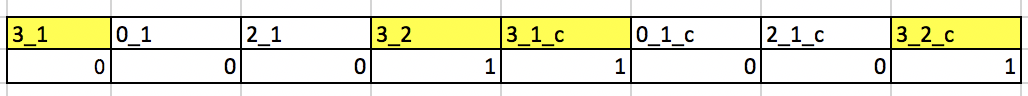guest_blog — Published On September 19, 2020 and Last Modified On December 29th, 2020

## Introduction

Supervised Contrastive Learning paper claims a big deal about supervised learning and cross-entropy loss vs supervised contrastive loss for better image representation and classification tasks. Let’s go in-depth in this paper what is about.Claim actually close to 1% improvement on image net data set¹.

Architecture wise, its a very simple network resnet 50 having a 128-dimensional head. If you want you can add a few more layers as well.

As shown in the figure training is done in two-stage.

• Train using contrastive loss (two variations)
• freeze the learned representations and then learn a classifier on a linear layer using a softmax loss. (From the paper)

The above is pretty self explanatory.

Loss, the main flavor of this paper is understanding the self-supervised contrastive loss and supervised contrastive loss.

As you can see from the above diagram¹ in SCL (supervised contrastive Loss), a cat is contrasted with any noncat. which means all cats belong to the same label and work as a positive pair and anything noncat is negative. This is very similar to triplet Data and how triplet loss² works.

In case you confused every cat images will be augmented also every-time so even from a single cat image we will have lots of cats.

Loss Function for supervised contrastive loss, although it looks monster it’s actually quite simple.

We will see some code later but first very simple explanation. every z is 128 dimensional vector which are normalised.

The loss function is with the assumption that every image has one augmentation, N images in a batch create a batch size = 2*N

Read the section of the paper “Generalisation to an arbitrary number of positives”¹

Numerator exp(zi.zj)/tau is a representation of all cats in a batch. Take dot product of zi which is the 128 dim vector of ith image representation with all the j^th 128 dim vectors such that their label is the same and i!=j.

The denominator is ith cat image is dotted with everything else as long it’s not the same cat image. Take the dot of zi and zk such that i!=k means it’s dotted with every image except itself.

Finally, we take the log probability and sum it overall cat images in the batch except itself and divide by 2*N-1

Total Loss sum of losses for all images

Code Let’s understand the above using some torch code.

Let’s assume our batch size is 4 and let’s see how to calculate this loss for a single batch.

For a batch size of 4, your input to the network will be 8x3x224x224 where I have taken image width and height 224.

The reason for 8 = 4X2 as we always have one contrast for each image, one needs to write a data loader accordingly.

The Super contrastive resnet will output you a dimension 8×128 lets split those properly for calculating the batch loss.

Numerator Code lets calculate this part

Anchor Dot Contrast in case you confused, our feature shapes are 8×128, lets take a 3×128 matrix and the transpose of that and dot them, see the below picture if you can visualize.

anchor_feature = 3×128 contrast_feature = 128×3 result is 3×3 as below

If you notice all diagonal elements are dot with themselves which we don’t want we will get rid of them next.

Linear Algebra fact if u and v are two vectors then u.v is maximum when u = v. So in each row if we take the max of anchor_dot_contrast and negate the same all diagonal will become 0.

Let’s drop the dimension from 128 to 2 to better see this and batch size of 1.

Mask. Artificial label creation and creating an appropriate mask for contrastive calculation. This code is a little tricky, so check the output carefully.

Let’s understand the output.The first row how to label 3 first image will be contrasted with others

Anchor dot contrast if you remember from above as below.

Loss again

Math recap

We already have the first part dot product divided by tau as logits.

I think that’s about the supervised contrastive loss. I think it’s very easy to understand the Self Supervised Contrastive Loss now which is simpler than this.

According to the paper, more contrast_count makes a better model which is self-explanatory. Need to modify the loss function for more than 2 contrast count, hope you can try it with the help of the above explanation.

## References